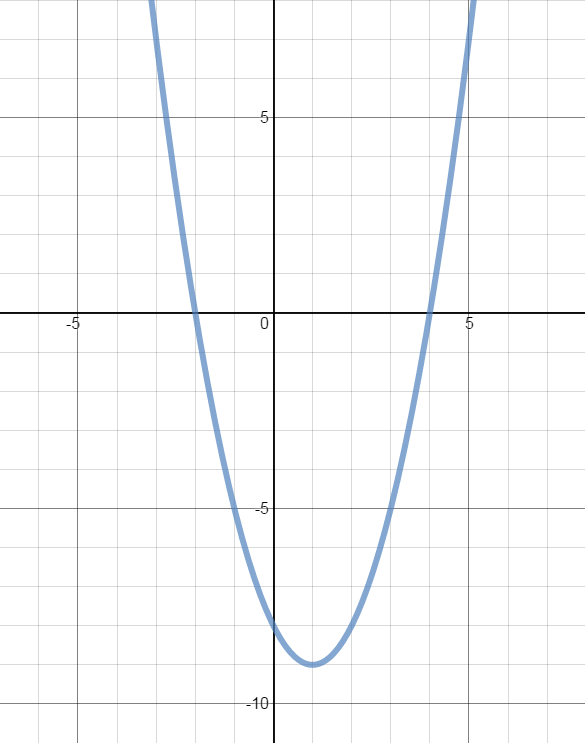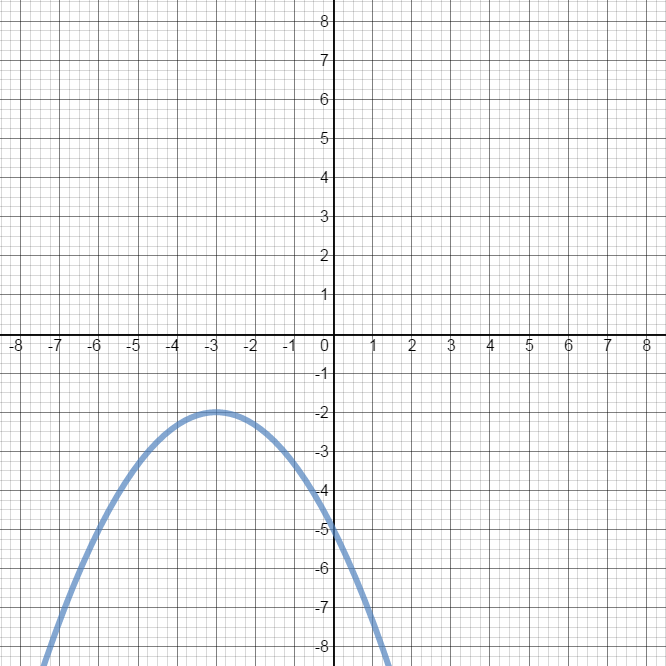#### Everything You Need in One Place

Homework problems? Exam preparation? Trying to grasp a concept or just brushing up the basics? Our extensive help & practice library have got you covered.#### Learn and Practice With Ease

Our proven video lessons ease you through problems quickly, and you get tonnes of friendly practice on questions that trip students up on tests and finals.#### Instant and Unlimited Help

Our personalized learning platform enables you to instantly find the exact walkthrough to your specific type of question. Activate unlimited help now!

0/10
##### Examples
###### Lessons
1. Determining the Characteristics of a Quadratic Function Using Various Methods

Determine the following characteristics of the quadratic function $y = -2x^2 + 4x + 6$:

• Opening of the graph

$y-$intercept

$x-$intercept(s)

• Vertex

• Axis of symmetry

• Domain

• Range

• Minimum/Maximum value

1. Using factoring
3. Using completing the square
4. Using the vertex formula
2. From the graph of the parabola, determine the:
• vertex
• axis of symmetry
• y-intercept
• x-intercepts
• domain
• range
• minimum/maximum value

1.2.3. Identifying Characteristics of Quadratic function in General Form: $y = ax^2 + bx+c$
$y = 2{x^2} - 12x + 10$ is a quadratic function in general form.

i) Determine:
• y-intercept
• x-intercepts
• vertex

ii) Sketch the graph.
1. Identifying Characteristics of Quadratic Functions in Vertex Form: $y = a(x-p)^2 + q$
$y = 2{\left( {x - 3} \right)^2} - 8$ is a quadratic function in vertex form.

i) Determine:
• y-intercept
• x-intercepts
• vertex

ii) Sketch the graph.
###### Topic Notes
Three properties that are universal to all quadratic functions: 1) The graph of a quadratic function is always a parabola that either opens upward or downward (end behavior); 2) The domain of a quadratic function is all real numbers; and 3) The vertex is the lowest point when the parabola opens upwards; while the vertex is the highest point when the parabola opens downward.